Click to Chat

1800-1023-196

+91-120-4616500

CART 0

• 0

MY CART (5)

Use Coupon: CART20 and get 20% off on all online Study Material

ITEM
DETAILS
MRP
DISCOUNT
FINAL PRICE
Total Price: Rs.

There are no items in this cart.
Continue Shopping• Complete JEE Main/Advanced Course and Test Series
• OFFERED PRICE: Rs. 15,900
• View Details

```Chapter 14: Compound Interest Exercise – 14.5

Question: 1

Ms. Cherian purchased a boat for Rs 16000. If the total cost of the boat is depreciating at the rate of 5% per year, calculate its value after 2 years.

Solution:Question: 2

The value of a machine depreciates at the rate of 10% per annum. What will be its value 2 years hence, if the present value is Rs 100000? Also, find the total depreciation during this period.

Solution:Question: 3

Pritam bought a plot of land for Rs 640000. Its value is increasing by 5% of its previous value after every six months. What will be the value of the plot after 2 years?

Solution: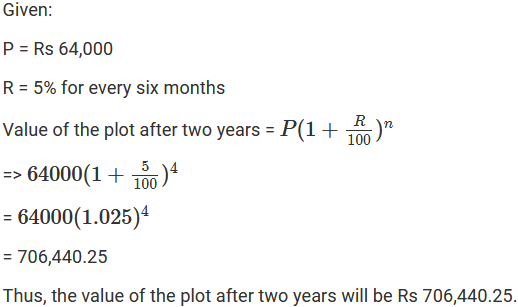Question: 4

Mohan purchased a house for Rs 30000 and its value is depreciating at the rate of 25% per year. Find the value of the house after 3 years.

Solution: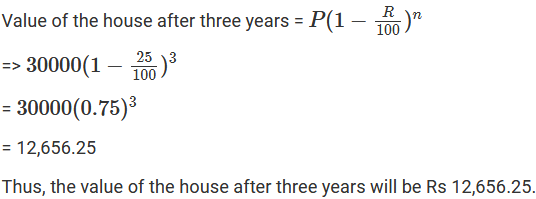Question: 5

The value of a machine depreciates at the rate of 10% per annum. It was purchased 3 years ago. If its present value is Rs 43740, find its purchase price.

Solution:Question: 6

The value of a refrigerator which was purchased 2 years ago, depreciates at 12% per annum. If its present value is Rs 9680, for how much was it purchased?

Solution: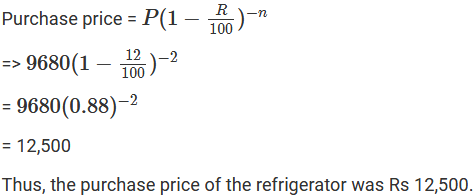Question: 7

The cost of a T.V. set was quoted Rs 17000 at the beginning of 1999. In the beginning of 2000 the price was hiked by 5%. Because of decrease in demand the cost was reduced by 4% in the beginning of 2001. What was the cost of the T.V. set in 2001?

Solution: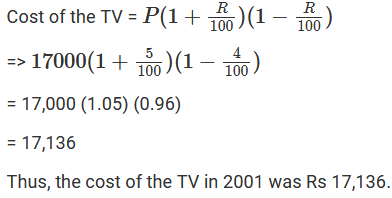Question: 8

Ashish started the business with an initial investment of Rs 500000. In the first year he incurred a loss of 4%. However during the second year he earned a profit of 5% which in third year rose to 10%. Calculate the net profit for the entire period of 3 years.

Solution: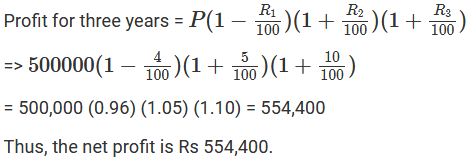```### Course Features

• 728 Video Lectures
• Revision Notes
• Previous Year Papers
• Mind Map
• Study Planner
• NCERT Solutions
• Discussion Forum
• Test paper with Video Solution